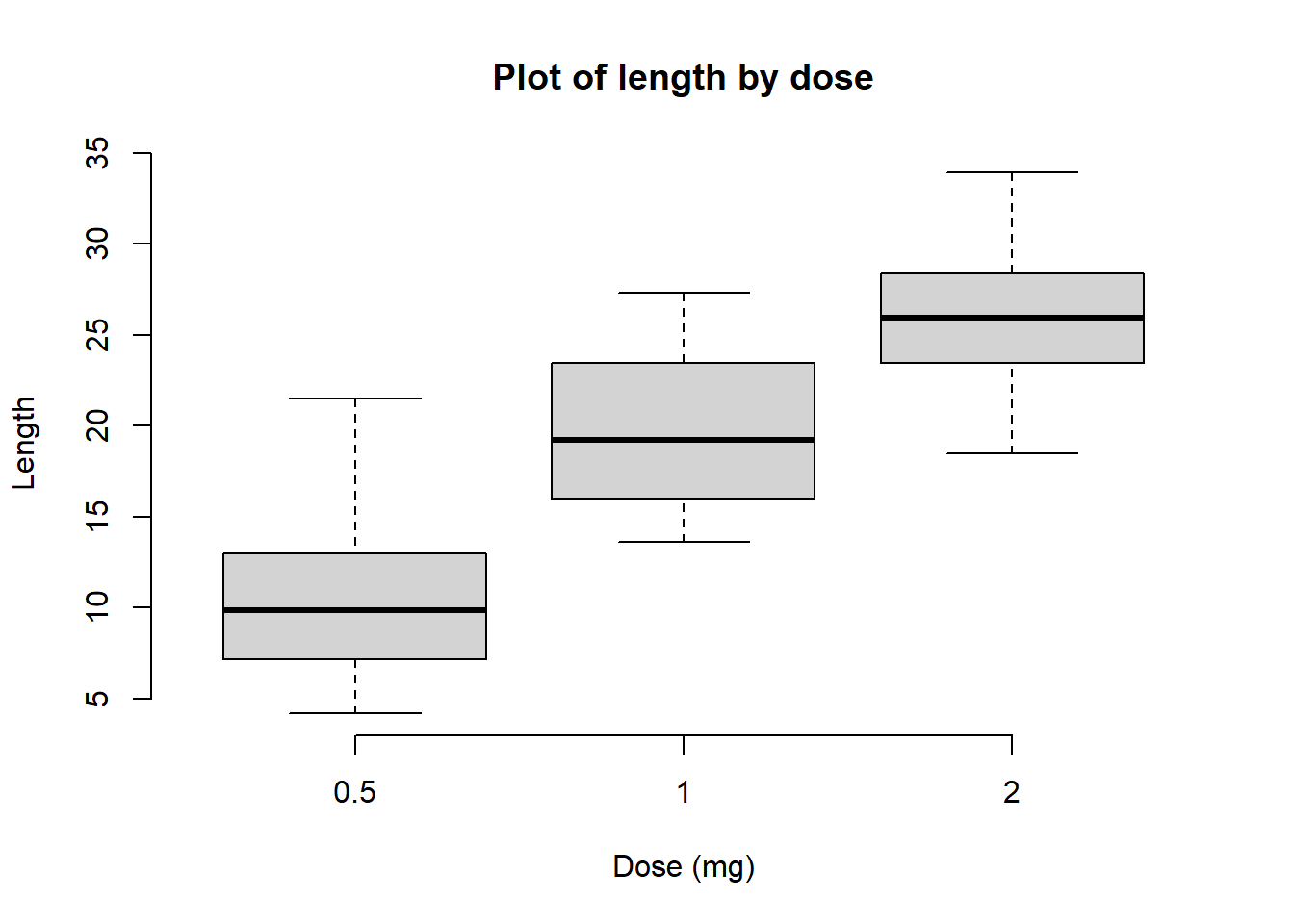We will use a dataset Tooth Growth.

The response is the length of (cells responsible for tooth growth) in 60 guinea pigs. Each animal received one of three dose levels of vitamin C (0.5, 1, and 2 mg/day) by one of two delivery methods, orange juice or ascorbic acid (a form of vitamin C and coded as VC).

# Print the first 6 rows
head(ToothGrowth, 6)
##    len supp dose
## 1  4.2   VC  0.5
## 2 11.5   VC  0.5
## 3  7.3   VC  0.5
## 4  5.8   VC  0.5
## 5  6.4   VC  0.5
## 6 10.0   VC  0.5
# Box plot of one variable
boxplot(ToothGrowth\$len)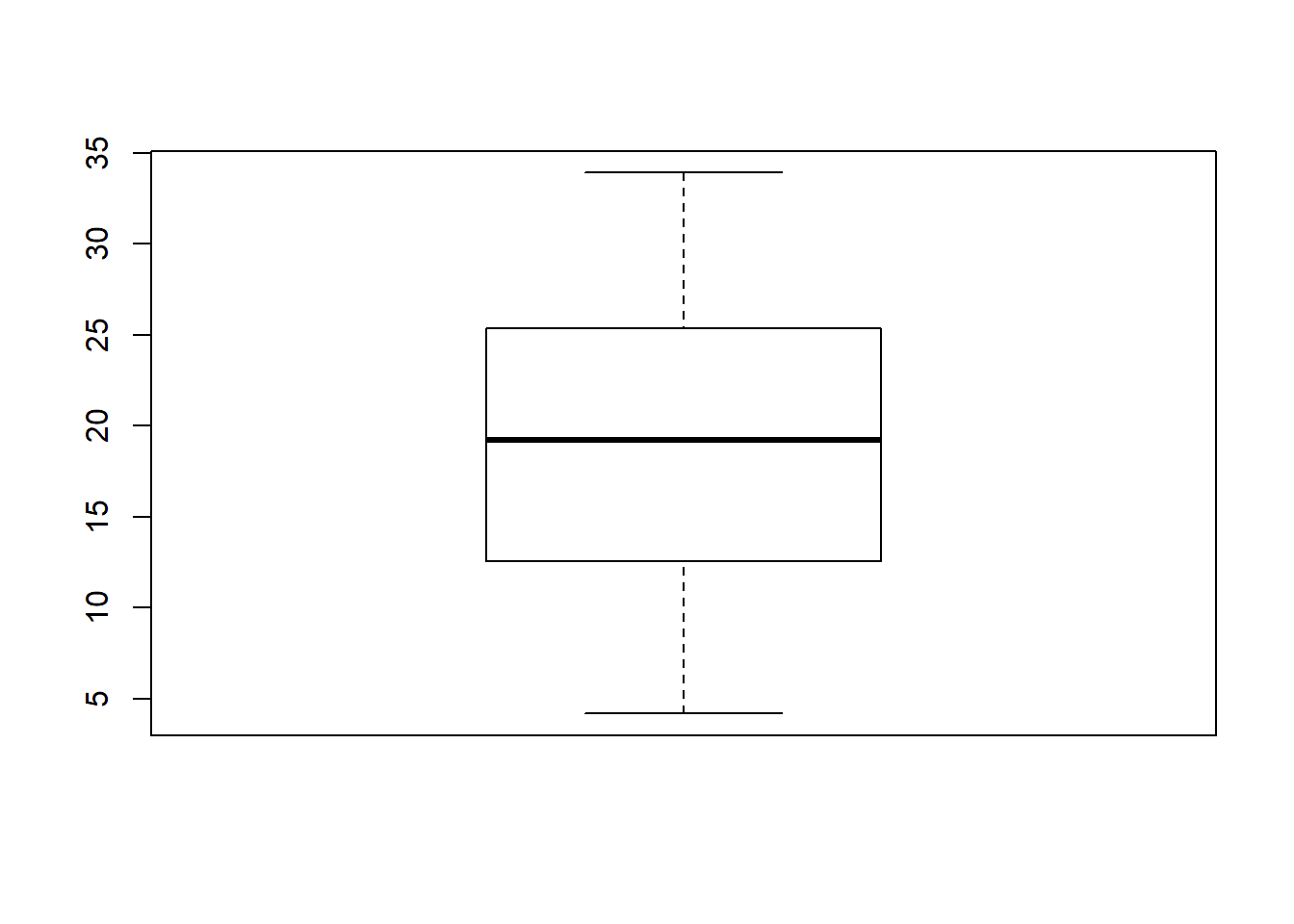## Box Plot By Group

# remove frame
boxplot(len ~ dose, data = ToothGrowth, frame = FALSE)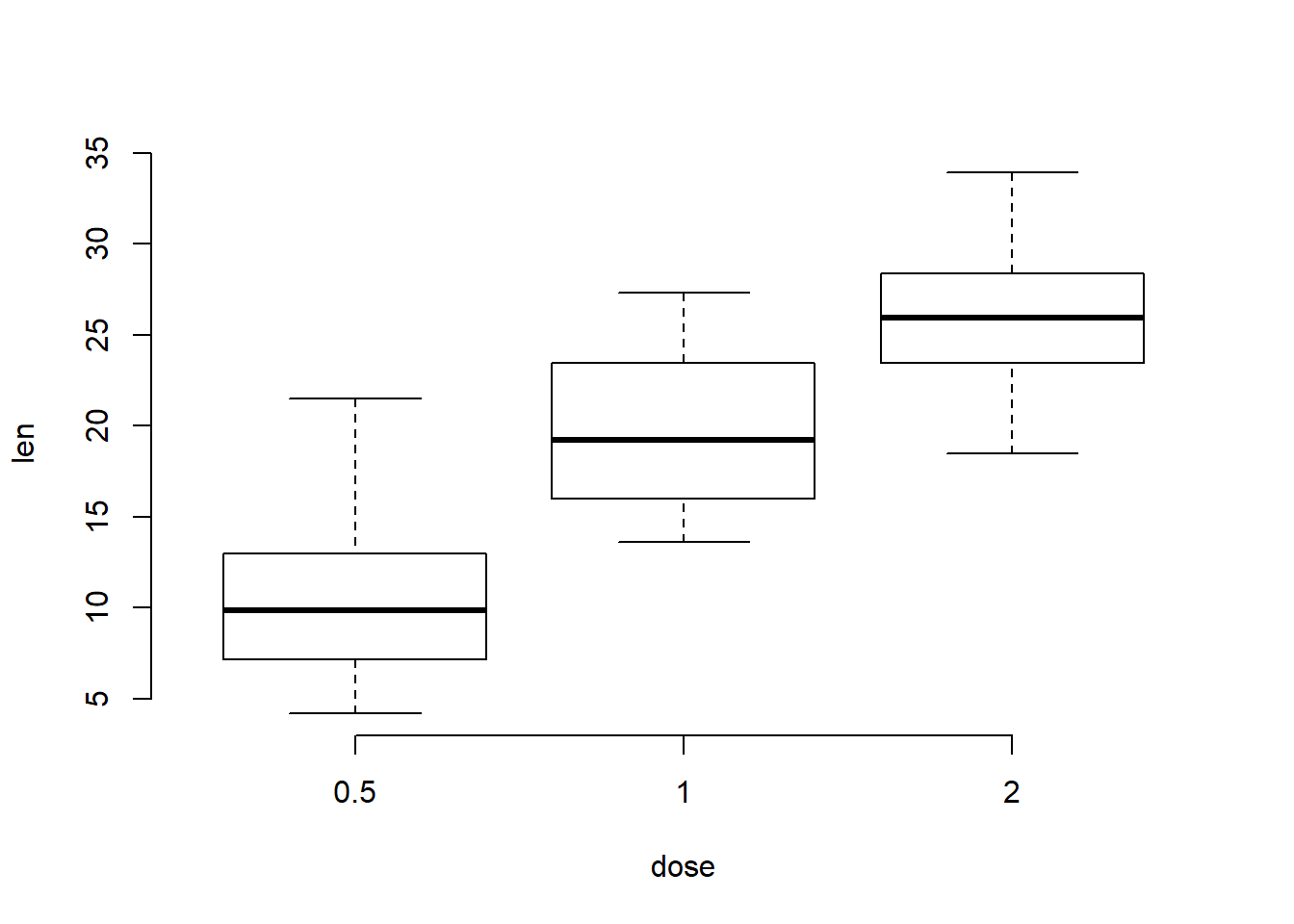# Horizontal box plots
boxplot(len ~ dose, data = ToothGrowth, frame = FALSE,
horizontal = TRUE)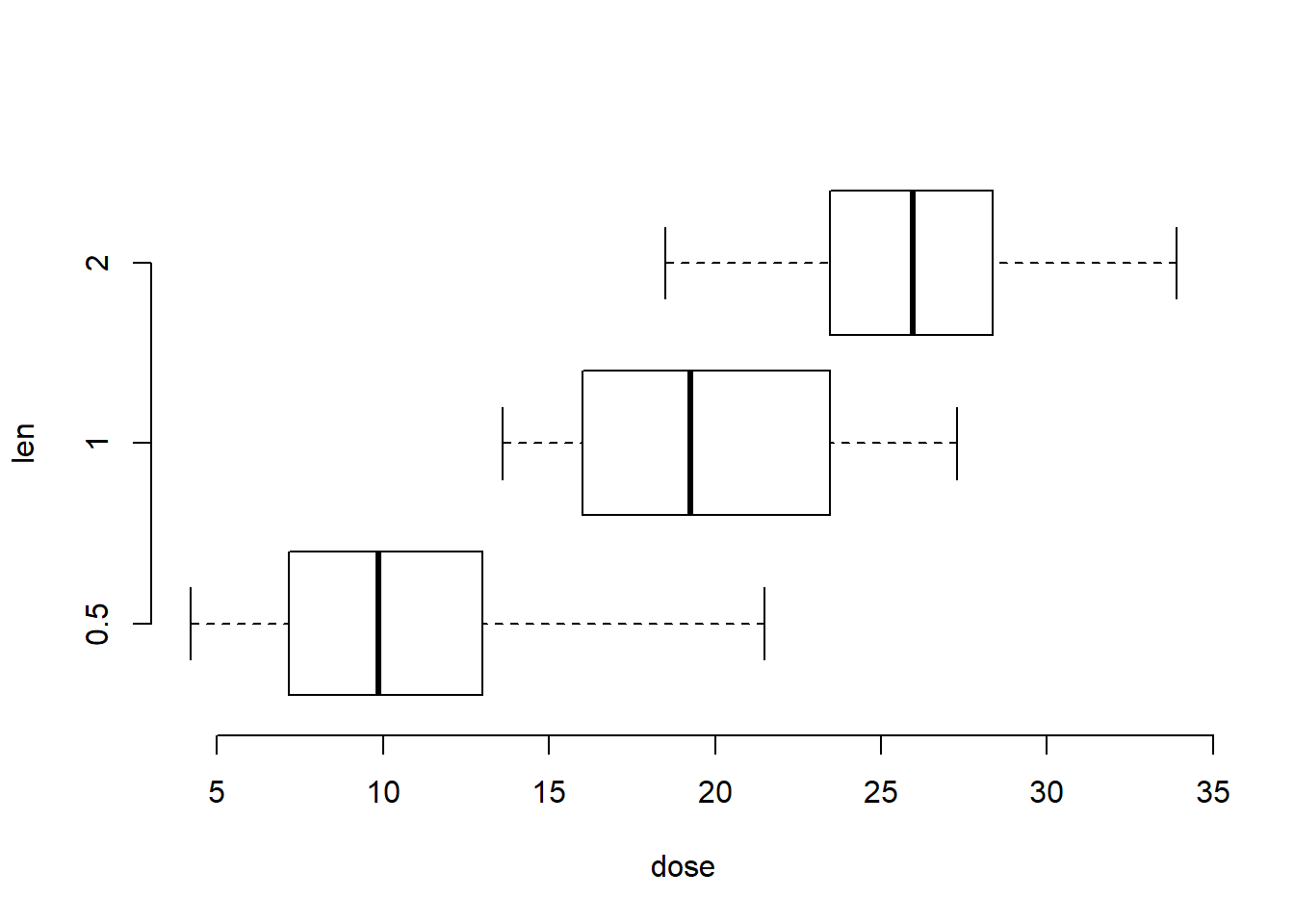# Notched box plots
boxplot(len ~ dose, data = ToothGrowth, frame = FALSE,
notch = TRUE)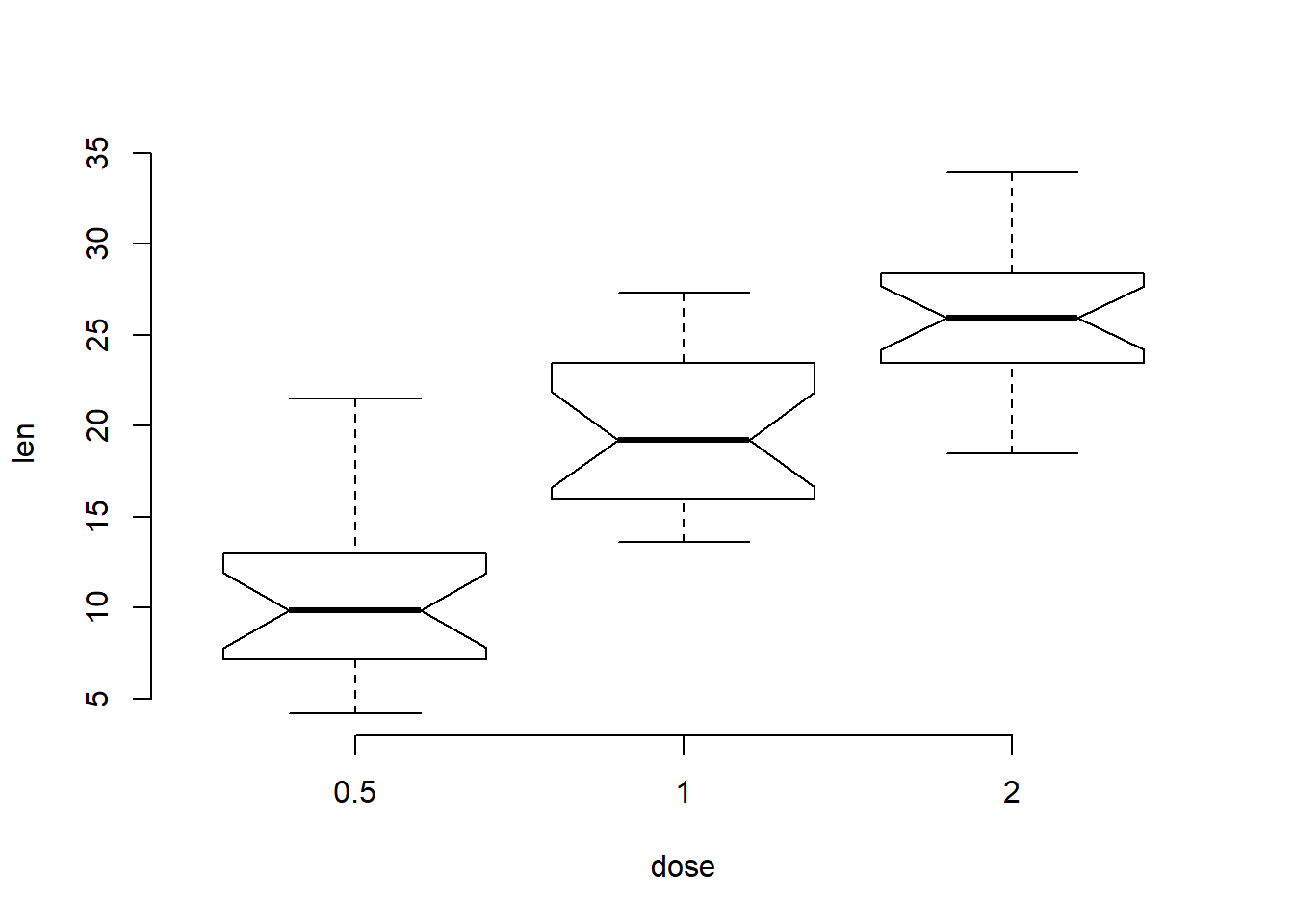Notch is used to compare groups. In the notched boxplot, if two boxes’ notches do not overlap this is “strong evidence” their medians differ (Chambers et al., 1983, p. 62).

#Change group names

boxplot(len ~ dose, data = ToothGrowth, frame = FALSE,
names = c("D0.5", "D1", "D2"))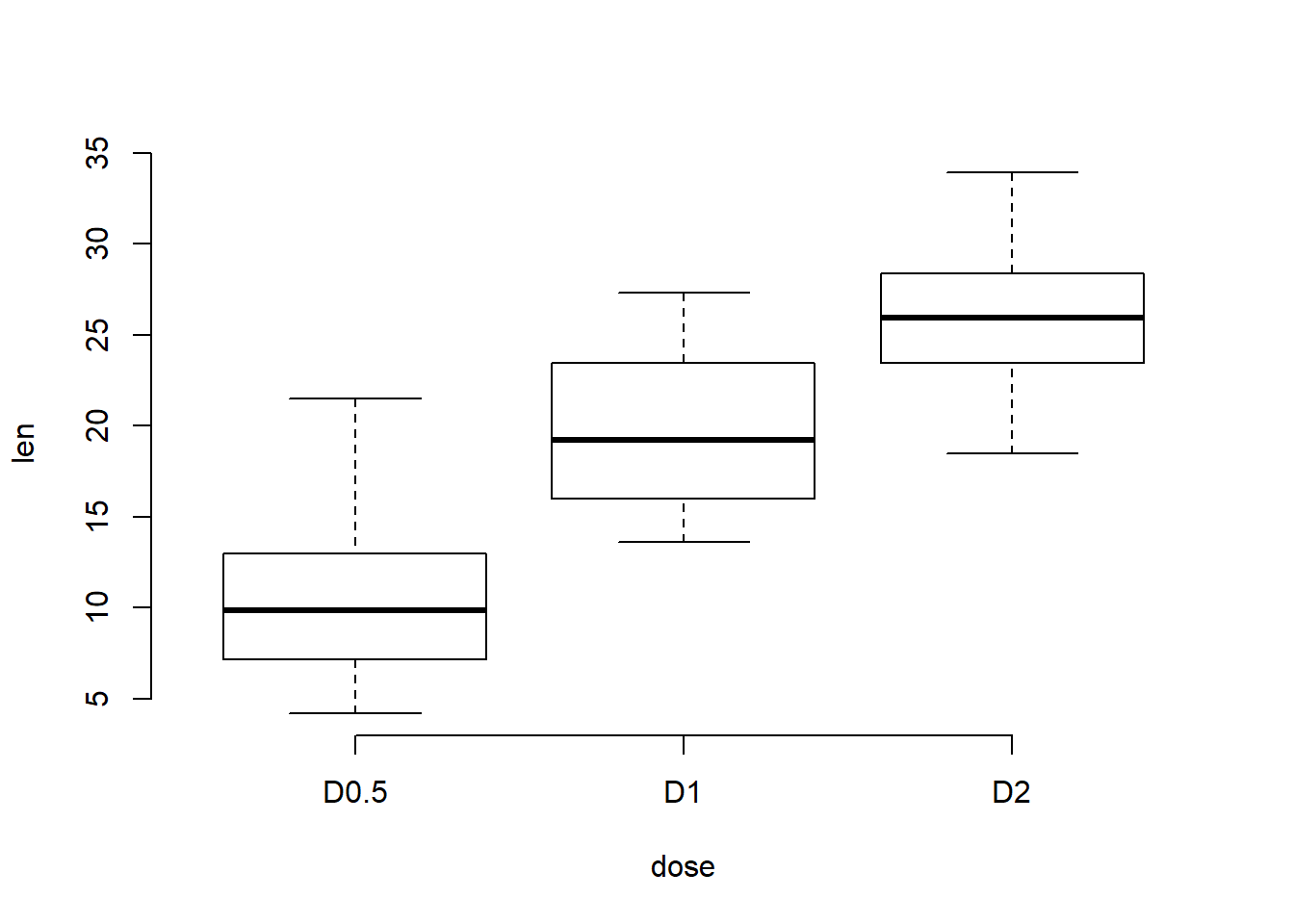## Change Color

# Change the color of border using one single color
boxplot(len ~ dose, data = ToothGrowth, frame = FALSE,
border = "steelblue")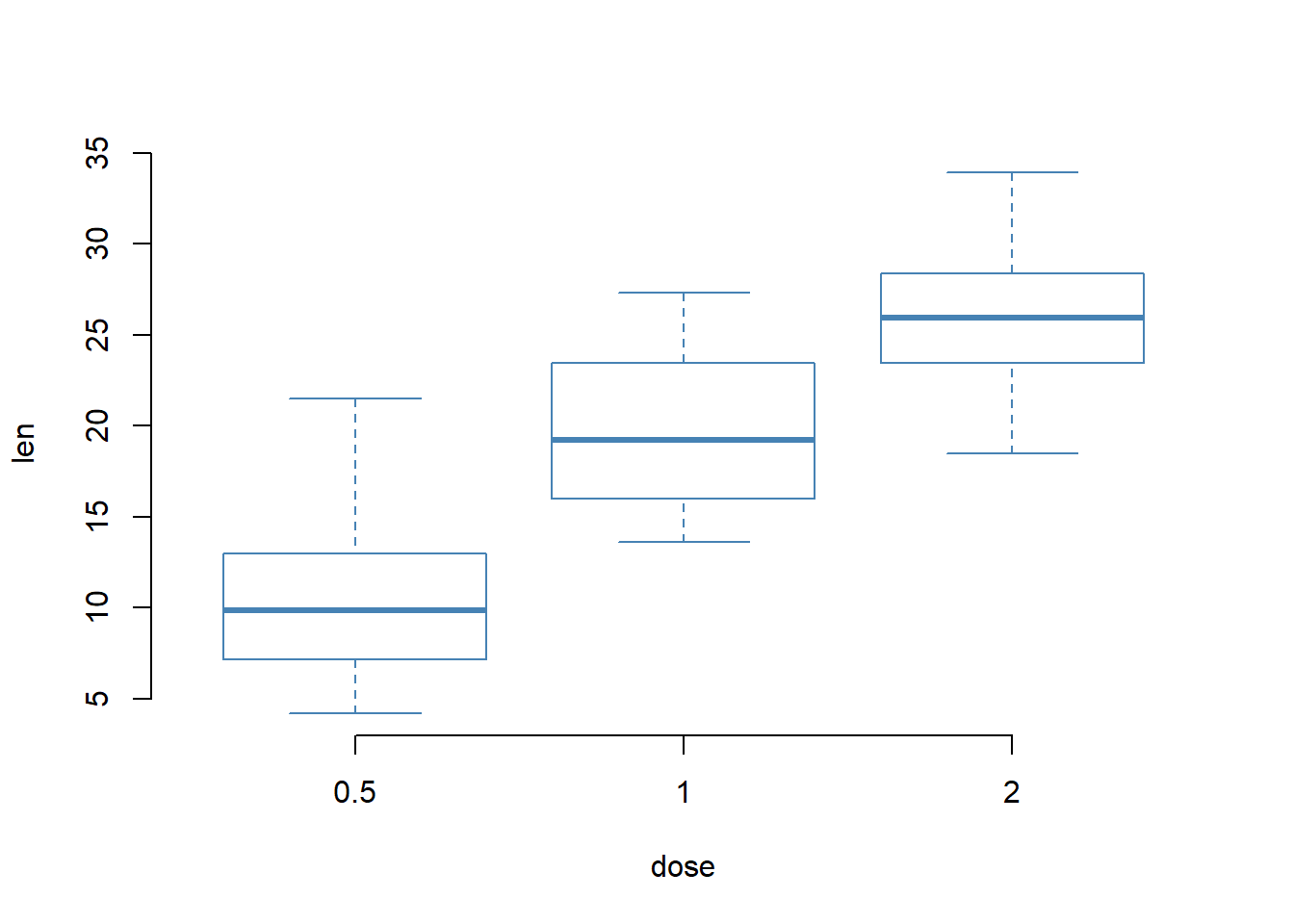#  Use different colors for each group
boxplot(len ~ dose, data = ToothGrowth, frame = FALSE,
border = c("#999999", "#E69F00", "#56B4E9"))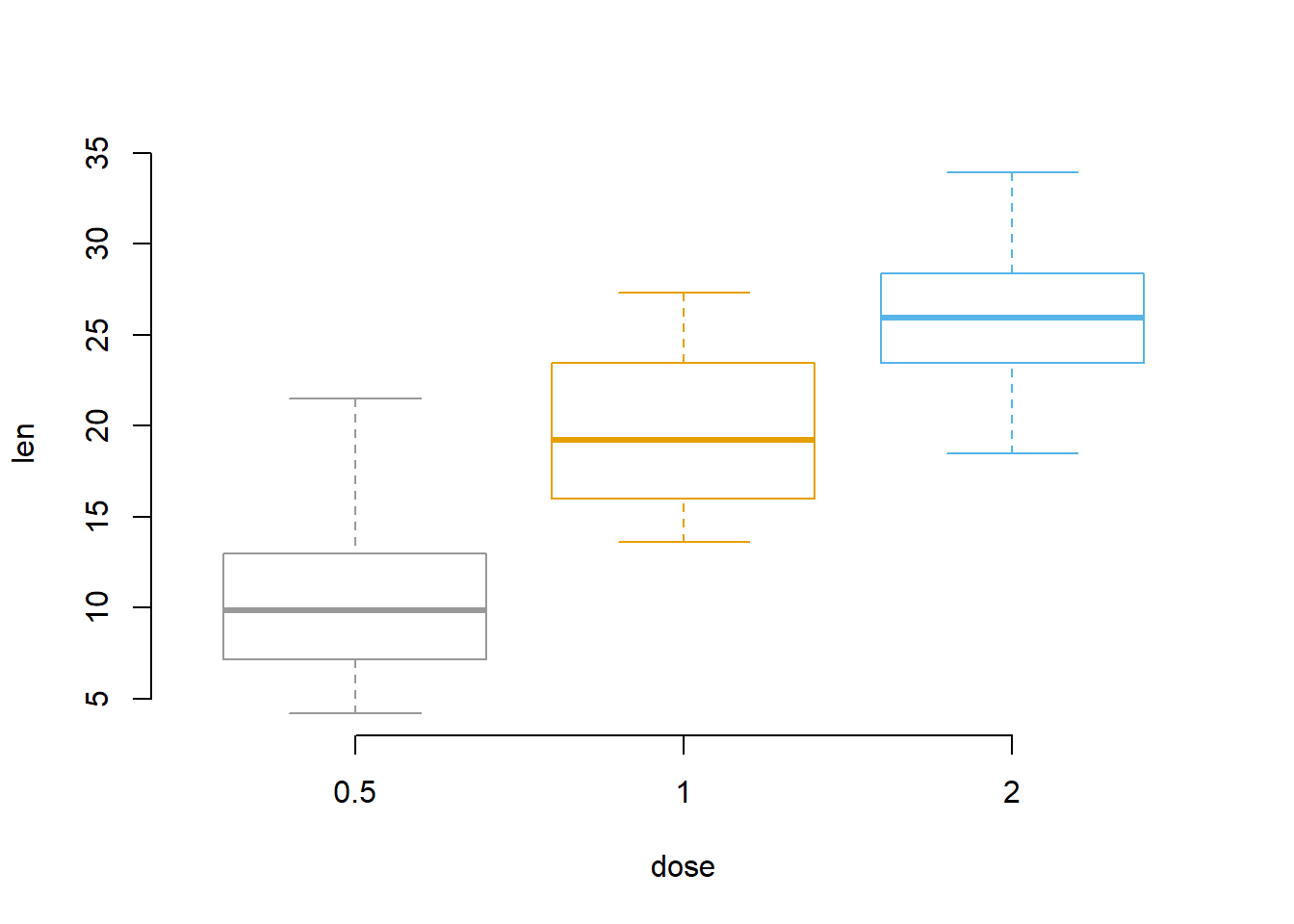# Change fill color : single color
boxplot(len ~ dose, data = ToothGrowth, frame = FALSE,
col = "steelblue")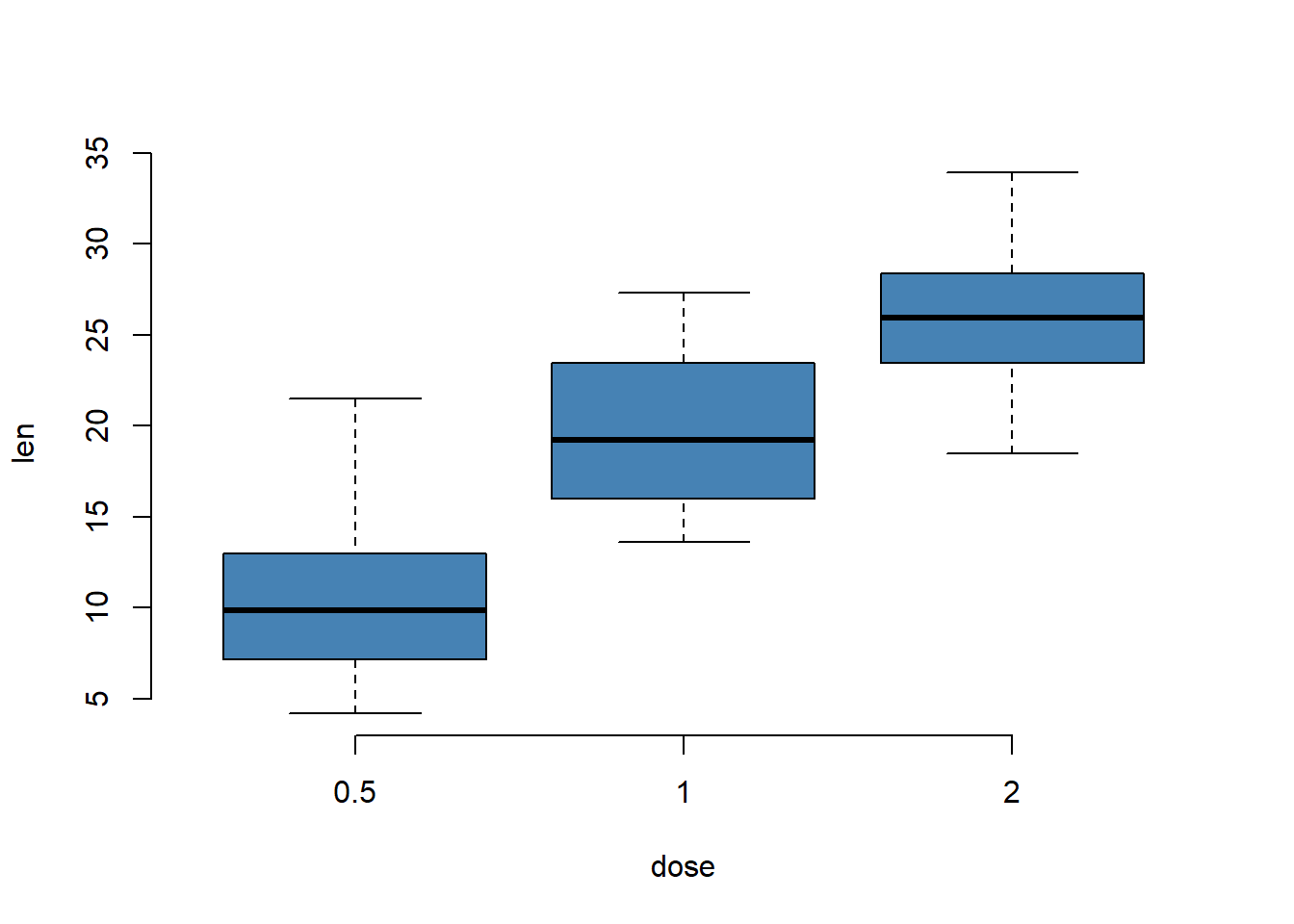# Change fill color: multiple colors
boxplot(len ~ dose, data = ToothGrowth, frame = FALSE,
col = c("#999999", "#E69F00", "#56B4E9"))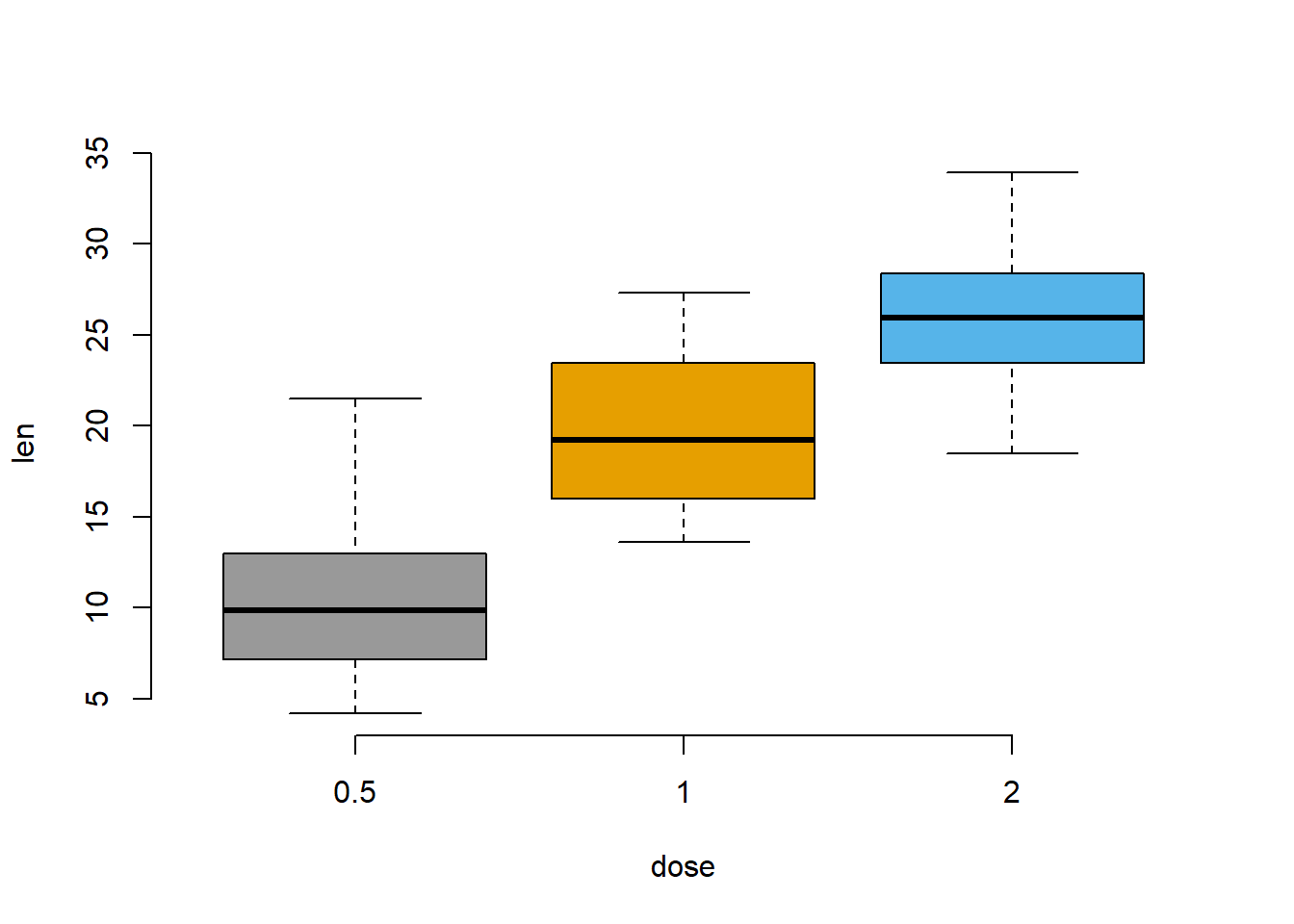## Box Plot with Multiple Groups

boxplot(len ~ supp*dose, data = ToothGrowth,
col = c("white", "steelblue"), frame = FALSE)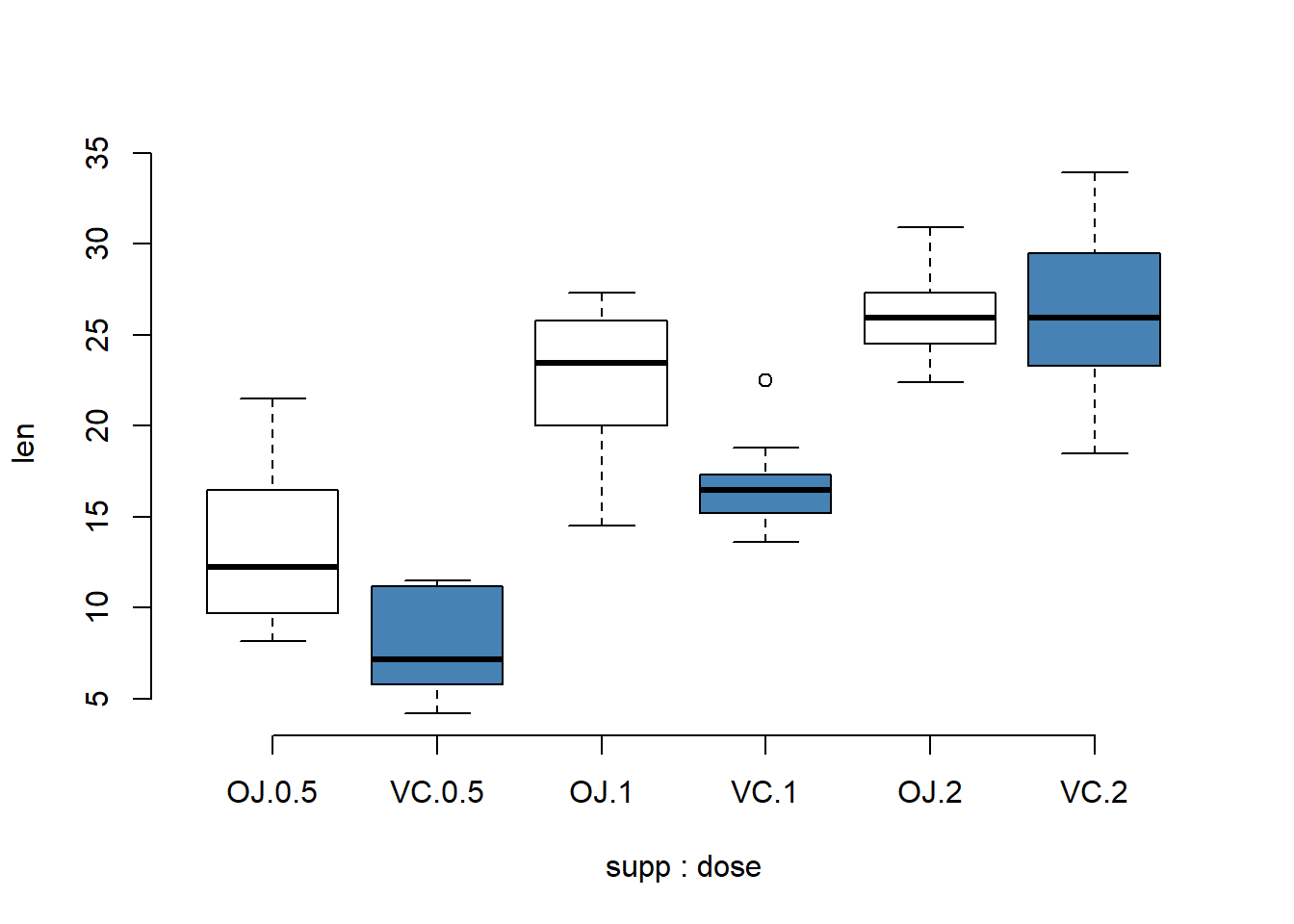## Change main title and axis labels

# Change axis titles
# Change color (col = "gray") and remove frame
boxplot(len ~ dose, data = ToothGrowth,
main = "Plot of length by dose",
xlab = "Dose (mg)", ylab = "Length",
col = "lightgray", frame = FALSE)